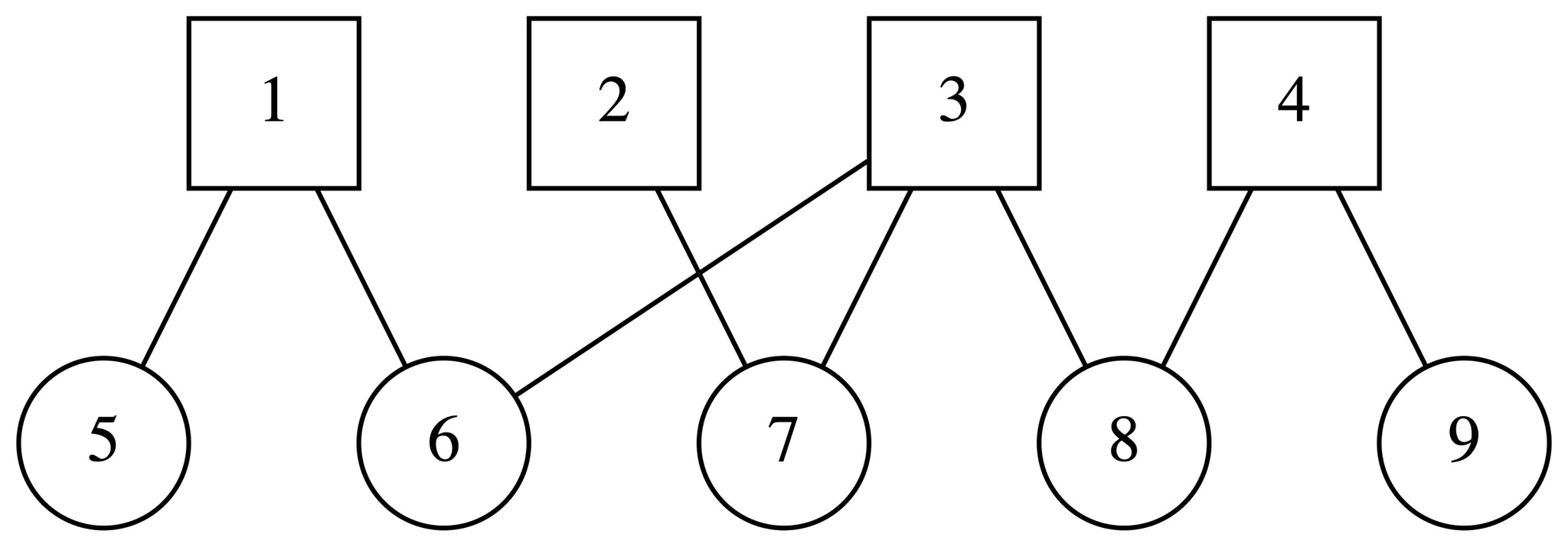시간 제한 메모리 제한 제출 정답 맞은 사람 정답 비율
3 초 512 MB 5 5 5 100.000%

## 문제

Alice and Bob obtained a map of the secret underground facility. The facility consists of $n$ security units and $m$ chemical labs, connected by bidirectional tunnels. The map of this facility forms a tree: there are exactly $n + m - 1$ tunnels, and there are no cycles. Vertices corresponding to security units have numbers from $1$ to $n$, chemical labs have numbers from $n+1$ to $n+m$. Each tunnel connects a security unit to a chemical lab; there are no tunnels between two security units or two chemical labs.

In case Alice or Bob gets captured, they decided to split the map into two pieces. To do that, they calculated the Prüfer code of the tree. Alice then saved some of the numbers between $1$ and $n$ to her data storage in the same order as they go in the original code, and Bob saved some of the numbers from $n+1$ to $n+m$ to his storage in the same way.

A Prüfer code of a tree on $k$ vertices is a sequence of $k - 2$ integers from $1$ to $k$, constructed as follows. Find the leaf (a vertex with degree one) with the smallest label, remove it from the tree, then print the label of its only neighbor. Repeat this $k - 3$ more times, until only one edge remains. The printed sequence of $k - 2$ vertex labels is the Prüfer code.

Alice and Bob safely returned and they are ready to combine their data to restore the original map. They could make a mistake during the backup, meaning no such map exists. Alice and Bob need your help to restore any possible map of the facility consistent with the collected data, so that both Alice's and Bob's parts are subsequences of the Prüfer code of the map.

## 입력

The first line of the input contains four integers $n$, $m$, $k_a$, and $k_b$ ($2 \le n, m \le 10^5$; $1 \le k_a, k_b$; $k_a + k_b \le n + m - 2$). The second line contains $k_a$ integers $a_1, a_2, \ldots, a_{k_a}$ ($1 \le a_i \le n$) --- Alice's part of the map. The third line contains $k_b$ integers $b_1, b_2, \ldots, b_{k_b}$ ($n + 1 \le b_i \le n + m$) --- Bob's part of the map.

## 출력

If there's no such map, print "No".

Otherwise, print "Yes" on the first line, followed by $n + m - 1$ lines describing the possible facility map. Each line should contain two integers $u_i$ and $v_i$ --- the security unit and the chemical lab connected by the $i$-th tunnel of the facility.

## 예제 입력 1

4 5 4 2
1 3 3 4
7 8


## 예제 출력 1

Yes
1 5
1 6
2 7
6 3
3 7
9 4
3 8
4 8## 예제 입력 2

4 3 3 1
3 2 2
6


## 예제 출력 2

No


## 노트

The Prüfer code of the tree in the first example is $(\underline{7}, \mathbf{1}, 6, \mathbf{3}, \mathbf{3}, \underline{8}, \mathbf{4})$.